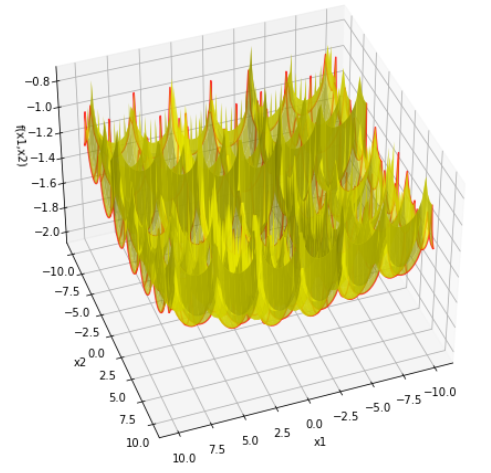top of page
Search

# Python Implementation of CROSS-IN-TRAY FUNCTION

Updated: Aug 5, 2021Mathematical DefinitionInput Domain

The function is usually evaluated on the square xi ∈ [-10, 10], for all i = 1, 2.

Global Minima

The four global minima are located at

f(x0) = -2.06261, at x0 = (1.3491,-1.3491) , (1.3491,1.3491), (-1.3491,1.3491), and (-1.3491,-1.3491)

Description and Features

Dimension: 2

This class defines the Cross-in-Tray global optimization problem. This is a multimodal

minimization problem.

Cross-in-tray function are complex multi-peak function which tend to make the algorithm fall

into local optimization, so that the real optimum value cannot be obtained, which is used to test

the ability of the improved algorithm to deal with falling into premature.

Cross-in-tray function has multiple global minima. The function on a smaller domain depicts its

characteristics ‘cross’.

The function is continuous.

The function is non-convex.

The function is defined on 2-dimensional space.

The function is multimodal.

The function is non-differentiable.

The function is non-separable.

Python Implementation

```% Please forward any comments or bug reports in chat
Copyright 2021. INDUSMIC PRIVATE LIMITED.THERE IS NO WARRANTY, EXPRESS OR IMPLIED. WE DO NOT ASSUME ANY LIABILITY FOR THE USE OF THIS PROGRAM. If software is modified to produce derivative works, such modified software should be clearly marked. Additionally, user can redistribute it and/or modify it under the terms of the GNU General Public License. This program is distributed in the hope that it will be useful, but WITHOUT ANY WARRANTY. See the GNU General Public License for more details.
% for any support connect with us on help.indusmic@gmail.com
% Author: Parakh Jain

import matplotlib.pyplot as plt
from mpl_toolkits import mplot3d
from numpy import*

def f(x1,x2):
a=fabs(100-sqrt(x1*x1+x2*x2)/pi)
b=fabs(sin(x1)*sin(x2)*exp(a))+1
c=-0.0001*b**0.1
return c
x1=linspace(-10,10,100)
x2=linspace(-10,10,100)
X1,X2=meshgrid(x1,x2)

def plotter(E,A):
fig=plt.figure(figsize=[12,8])
ax=plt.axes(projection='3d')
ax.plot_surface(X1,X2,f(X1,X2),color='yellow',alpha=0.7)

ax.plot_wireframe(X1,X2,f(X1,X2), ccount=10,rcount=10,color='red, alpha=0.8)
ax.view_init(elev=E,azim=A)
ax.set_xlabel('x1')
ax.set_ylabel('x2')
ax.set_zlabel('f(x1,x2)')
plt.show()

from ipywidgets import interactive
iplot=interactive(plotter,E=(-90,90,5),A=(-90,90,5))
iplot```

References:

 Jamil, Momin, and Xin-She Yang. "A literature survey of benchmark functions for global optimization problems." International Journal of Mathematical Modelling and Numerical Optimization 4.2 (2013): 150-194.